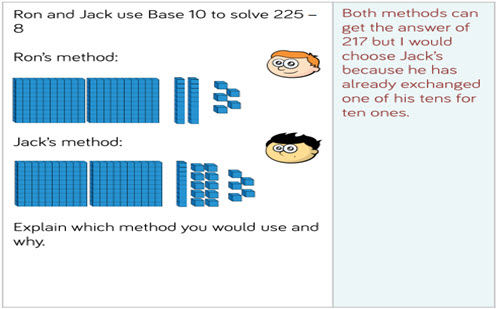# Addition and subtraction: numbers to 100 and 1,000

This guide provides a sequence of steps for exploring and developing addition and subtraction strategies, including an emphasis on not crossing 10s and 100s and crossing 10s and 100s.

Year level(s) Year 2, Year 3, Year 4, Year 5, Year 6
Audience Teacher
Purpose Teaching resource, Teaching strategies
Teaching strategies and pedagogical approaches Questioning, Concrete Representational Abstract model, Explicit teaching
Keywords add, subtract, addition, subtraction, multiples of 100, bar model, part-whole model, reasoning, problem-solving, number tracks,, calculations, place value, place value grid, 2-digit numbers, 3-digit numbers, base 10 blocks, explicit teaching

## Curriculum alignment

Curriculum connections Numeracy
Strand and focus Number, Build understanding
Topics Addition and subtraction, Place value, Operating with number
AC: Mathematics (V9.0) content descriptions
AC9M2N04
Add and subtract one- and two-digit numbers, representing problems using number sentences and solve using part-part-whole reasoning and a variety of calculation strategies

AC9M3A02
Extend and apply knowledge of addition and subtraction facts to 20 to develop efficient mental strategies for computation with larger numbers without a calculator

AC9M4N06
Develop efficient strategies and use appropriate digital tools for solving problems involving addition and subtraction, and multiplication and division where there is no remainder

Numeracy progression Additive strategies (P7, P8)
Number and place value (P5, P7)
Number patterns and algebraic thinking (P3)
Multiplicative strategies (P7)